Refer to our Texas Go Math Grade 1 Answer Key Pdf to score good marks in the exams. Test yourself by practicing the problems from Texas Go Math Grade 1 Lesson 3.2 Answer Key Add Tens and Ones.

Essential Question
How can you odd tens and ones?
Explanation:
Add tens place to tens place and ones place to ones

Explore
Choose a way to show the problem. Draw a quick picture to show your work.

For The Teacher

• Read the following problems. Barb has 20 pennies. Ed has 3 pennies. How many pennies do they have? Kyle has 40 pennies. Kim has 5 pennies. How many pennies do they have?

Math Talk
Mathematical processes

Model and Draw

How can you find 30 + 4?
___ tens ___ ones
30 + 4 = ____
Explanation:3 tens 4 ones
30 + 4 = 34

Share and Show

Use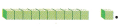Write how many tens and ones. Draw to show tens and ones. Write the sum.

Question 1.
60 + 6 = ___
___ tens ___ ones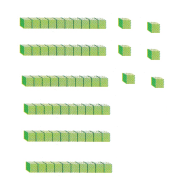Explanation:
60 + 6 = 66
6 tens 6 ones

Question 2.
50 + 3 = ___
___ tens ___ ones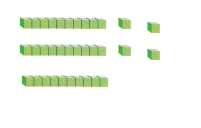Explanation:
50 + 3 = ___
___ tens ___ ones

Question 3.
90 + 8 = ___
___ tens ___ onesExplanation:
90 + 8 = 98
9 tens 8 ones

Question 4.
20 + 1 = ___
___ tens ___ ones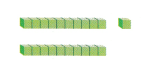Explanation:
20 + 1 = 21
2 tens 1 ones

Problem Solving

Write how many tens and ones.
Draw to show tens and ones. Write the sum.

Question 5.
40 + 2 = ___
___ tens ___ ones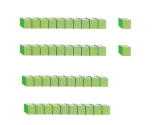Explanation:
40 + 2 = 42
4 tens 2 ones

Question 6.
70 + 9 = ___
___ tens ___ ones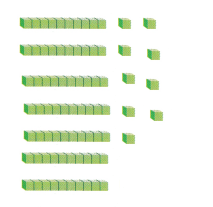Explanation:
70 + 9 = 79
7 tens 9 ones

Draw to show tens and ones. Write the addition sentence.

Question 7.
Luke has 30 blocks. Elle has 6 blocks. How many blocks do they have?
___ + ___ = ___
__ blocksExplanation:
30 + 6 = 36
36 blocks

Question 8.
H.O.T. Penny has 4 boxes of 10 movies. Nick has 8 movies. How many movies do they have?Explanation:
Penny has 4 boxes of 10 movies.
4 x 10 = 40
Nick has 8 movies.
40 + 8 = 48
48 movies that they have

Question 9.
Multi-Step Ryan has 2 boxes of 10 games. Ian has 3 boxes of 10 games. Then Mindy gives Ryan 5 more games. How many games do they have now?
___ + ___ = ___
__ games
2 x 10 = 20
3 x 10 = 30
30 + 20 + 5 = 55
Explanation:
50 + 5 = 55
55 games

Question 10.
Lara planted two pepper plants. One grew 10 peppers. The other grew 6. Which number sentence shows how many peppers grew in all?(A) 10 – 6 = 4
(B) 1 + 6 = 7
(C) 10 + 6 = 16
Explanation:
10 + 6 = 16
1 tens and 6 ones

Question 11.
Multi-Step Karl has 2 bags of 10 red marbles, 1 bag of 10 green marbles, and 8 blue marbles. How many marbles does Karl have?
(A) 31 marbles
(B) 38 marbles
(B) 28 marbles
Explanation:
2 x 10 = 20
1 x 10 = 10
20 + 10 + 8 = 38

Question 12.
Use Tools Which shows 30 + 5 = 35?Explanation:
3 tens blocks and 5 ones blocks

Question 13.
Texas Test Prep What is the sum? 50 + 7 = ?
(A) 7
(B) 57
(C) 52
Explanation:
50 + 7 = 57
5 tens 7 ones

Take Home Activity

• Ask your child to explain how to find the sum of 20 + 7.
Explanation:
20 + 7 = 27

### Texas Go Math Grade 1 Lesson 3.2 Homework and Practice Answer Key

Write how many tens and ones. Draw to show tens and ones. Write the sum.

Question 1.
30 + 7 = ___
___ tens __ ones
3 tens 7 ones
Explanation: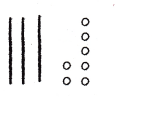Lines represent 10 and dot represent 1

Question 2.
90 + 3 = ___
___ tens __ ones
9 tens 3 ones
Explanation:
Lines represent 10 and dot represent 1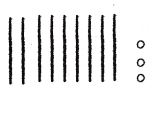Problem Solving

Draw to show tens and ones. Write the addition sentence. Write the sum.

Question 3.
Multi-Step Jeff makes 4 stacks of 10 books. Max makes 4 stacks of 10 books. Then Josh gives Jeff 8 more books. How many books do they have?
___ + __ = ___
___ books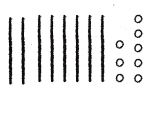Explanation:
Jeff makes 4 stacks of 10 books = 4 x 10 = 40 books
Max makes 4 stacks of 10 books = 4 x 10 = 40 books
Josh gives Jeff 8 more books = 40 + 8 = 48 books
48+40 = 88 books total they have

Texas Test Prep

Question 4.
Mark drew two castles. One had 10 rooms. The other had 7 rooms. Which number sentence shows how many rooms he drew in all?
(A) 10 + 7 = 17
(B) 10 – 7 = 3
(C) 1 + 7 = 8
Explanation:
One had 10 rooms. The other had 7 rooms. 10 + 7 = 1 tens + 7 ones = 17

Question 5.
Which shows 60 + 2 = 62?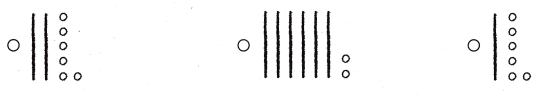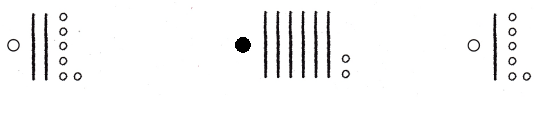Explanation:
each line represents 10 and circle represents 1
60 + 2 = 6 Tens + 2 Ones= 62

Question 6.
What is the sum?
40 + 6 = ___
(A) 46
(B) 42
(C) 64
Explanation:
40 + 6 = 4 Tens + 6 Ones = 46

Question 7.
Multi-Step Tim has 1 box of 10 colored pencils. Sam has 4 boxes of 10 pencils. Then Joey gives Tim 3 more pencils. How many pencils do they have?(A) 43
(B) 5
(C) 53